﻿ Day-Ahead Price Forecasting of Electricity Market Using<i> </i>Neural Networks and Wavelet TransformElectrical and Electronic Engineering

p-ISSN: 2162-9455    e-ISSN: 2162-8459

2018;  8(2): 37-52

doi:10.5923/j.eee.20180802.02### Day-Ahead Price Forecasting of Electricity Market UsingNeural Networks and Wavelet Transform

Kamran Rahimimoghadam1, S. Hossein Hosseinian1, M. Saravi2

1Faculty of Engineering, Amir Kabir University, Iran

2Emeritus Professor of Islamic Azad University of Iran

Correspondence to: M. Saravi, Emeritus Professor of Islamic Azad University of Iran.
 Email: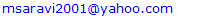Abstract

In a competitive environment, participants chooses their bid with regard to policy their advantages and market conditions. Therefore one of the essential and necessary discussions in competitive environment is prices prediction. In this paper artificial neural network method is used for load prediction by considering the most maximum impact factors in prediction and most influencing data as input of model. Proposed model is experienced on Nord Pool electricity market and the results are checked in various stages. Also for expression of system error, an indicator of MAPE has been used. This error provides a good indication of the constraints and applicability of these predictions. To reduce the size of the input data and obtain better results, a filter been used to separate parameters with similar frequency. In addition to the electrical loads, the daily temperature has been used as a factor for forecasting to achieve better results. The MAPE obtained from the load forecasting results confirm that the proposed technique is robust in forecasting future load demands and provides reliable forecasts for the daily operational planning of Nord Pool market.

Keywords: Price Forecasting, Wavelet Transform, Series Analysis, Electricity Markets

Cite this paper: Kamran Rahimimoghadam, S. Hossein Hosseinian, M. Saravi, Day-Ahead Price Forecasting of Electricity Market Using Neural Networks and Wavelet Transform, Electrical and Electronic Engineering, Vol. 8 No. 2, 2018, pp. 37-52. doi: 10.5923/j.eee.20180802.02.

### 1. Introduction

The beginning of the recent reforms in electricity industries start from 1970. Since 1970 small and new supplier were allowed to have activity in the electricity power generation sector. In 1978 the U.S. government approved a law according to which the electricity power companies were forced to buy electricity from such supplier. The electricity markets in England and Wales, which is called POOL, was formed in 1990 and its operational mechanism is one of the best mechanisms in the world. Followed by Norway in 1991, competitive markets such as POOL were designed and in 1996 this market extended in Sweden. Now this market is known as NORD POOL. In 1998 the Australian National Electricity Market was launched. The competing exchange of electrical energy in Spain also began in 1998 . For next day electricity price forecasting, reference  used dynamic regression and transfer function according to the analysis of time series. These models were based on high frequency variations, the mean and variance of seasonal factors and seasonal effects over a weekend. Input function in this model is the white noise, and the model assumptions must be completely clear. The model parameters will be estimated from the assumptions, and if the assumptions are not accurate, the system will not provide any answer. Moreover, in this method some simplifying hypotheses have been considered such as elimination of intense fluctuations of price that are not very realistic or accurate. Besides of the price of supply and demand was assumed to be constant. If the request was different, prediction would contain error and its standard deviation would be greater. Also transfer function model would involve more calculations, so for about five minutes would be necessary to estimate and predict. In  Arima model was used for prediction of electricity markets in Spain and California. The mean error of the prediction for the Spanish market was 10% and 11% for California. In this model, the variables were load and price and there was no special attention to other influencing variables in prediction. In  Wavelet and Arima procedures are combined to predict. In this method the electricity price time series has been split into several time series with the better being the Wavelet method.
Each of the components has been predicted individually by Arima method and finally the results have been transformed by inverse wavelet and have been combined to predict the final outcome. In this model the data of the Spanish electricity market have been used and shown that because of the smoothness properties of the wavelet transform. The prediction errors of Arima depend on the approach that uses only Arima. In this approach other influencing parameters on price have been ignored because they are not appropriate for the Arima model. Indeed, due to the strategies of price proposal, time series of price has a non-periodic oscillatory nature. He proposed a set of dynamic models that are linked to each other such as Markov chain. Also, the specific kind of Markov model named Iohmm was used for analysis and prediction of instantaneous price of energy. Arima composed two artificial neural networks and was able to predict electricity price accurately as well as predict some of the dynamic information such as market behavior and its effect on future prices. The method was implemented on the Spanish electricity market. According to the results, it was obvious that in accordance to the other methods, predicted error in this method was not fairly well. Other work in price prediction using the artificial neural network with Wavelet transform can be found in . In this work, the inputs were previous load and previous price, and were experienced in the PJM market. In proposed model the error was partly high, e.g., 8.9% for summer and 9.59% for winter.  used a combination of neural-fuzzy or ANFIS to predict the future MCP of Ontario’s market. The data for this prediction came from previous MCP of market, time clock system, sharing system operation and transmission constraints. The data for training the neural network was from 2-4 previous weeks. The data and training algorithm used the Levenberg- Marquardt algorithm. Other methods for prediction is the new method of fuzzy-neural having inner layer with a new mechanism which is a multi-dimensional learning algorithm combined with fuzzy logic and a special education model for non-stationary behavior, as well as mutations of electricity prices . The results of this prediction show that it is better than the previous methods such as Arima and RBF.
Despite the ongoing investigation in the load and price prediction area, most of the existing works do not take into account some information like weather changes and predicted load, also the prediction error is not fairly well. Therefore in this study it has tried to address the short-term prediction of electricity price using Neural Network with the method of Wavelet Transform and the Time Series of sensitivity analysis using Cross-Correlation or Autocorrelation on input data. This model has been evaluated in the Nord Pool electricity market and the results of each season obtained in following three stages: first without considering the weather changes, second with considering the weather changes, and then with considering predicted load and temperature. To reduce the size of the input data to the neural network and to obtain better results, a filter used to separate parameters with similar frequency. The rest of the paper is organized as follows: Section 2 described factors and limitation, and section 3 Neural Network is going to be explained. Section 4 presents sensitivity analysis, and in Section 5, 6 the load forecasting process and price forecasting are evaluated. Section7 contain results and discussions; finally, this paper is concluded in Section 8.

### 2. Factors and Limitations

#### 2.1. The Factors in Electricity Price Prediction

The factors in electricity price prediction can be summarized as:
(i)-Time: hours, day, days of week, month, year and specific days
(ii)-Price: previous price
(iv)-Temperature: wind speed, humidity that has direct impact on temperature and consumption
(v)-Other utility parameters

#### 2.2. Problems of Electricity Price Forecasting

One of the biggest challenges in price prediction is instability of electricity generation and consumption; the main causes of instability are strategies when selected by market operators, and the large uncertainties affecting the price of electricity . Other problems in electricity price prediction can be described as: lack of access to the important information and factors on electricity price such as lack of accurate information about supplier and customer constraints, as well as lack of access to the hourly temperature and other influencing factors. So lack of clear recognition of input data for price forecasting can be counted as a problem for price forecasting.
Short term prediction of load in power systems have many applications such as: production scheduling, improving the reliability of power systems, exploit market information and load distribution, planning to provide fuel for power stations, exiting and entering of units, transformers, transmission lines and distribution networks and load levelling [9, 10].

### 3. Neural Network

Recent research activities in artificial neural networks (ANNs) have shown that ANNs have powerful pattern classification and pattern recognition capabilities. A neural network is a concept in computing which is used to get the mathematical model, and the concept is similar to the structure and operation of the brain. It consists of a number of simple processing units ‘cells’ or ‘nodes’. When a given set of cells (the inputs) are stimulated, the signals are passed through the network from node to node and finally exit from the network through another set of simplified nodes (the outputs) . The computational elements or nodes in the concealed and output layers are generally nonlinear. The simple node sums ‘N’ weighted inputs are multiplied with a weight of neural network and then is passed through the tanh activation function. This is commonly used in training the non-linear function. The node is characterized by an internal threshold or bias and by the type of non-linearity. There are three common types of nonlinearities: hard limiters, threshold logic elements and sigmoidal nonlinearities. More complex nodes may include temporal integration or other types of time dependencies and more complex mathematical operations than summation .

#### 3.1. Structure of Proposed Neural Network

The proposed neural network is non-linear Multi-Layer Perceptron and has an input layer with four groups of information. Price of previous hours, amount of consumed charge during different hours, time of mentioned charge and price, hourly temperature and the numbers of layers in the network are obtained by testing to achieve the best results. Finally an output layer will be obtained in which the price of the next hours can be set from next 1-168 hour(s). The function of selected performance of neurons in the middle layer is the tangent sigmoid function and corresponds to the output layer of linear function network. Also, the training algorithm is back propagation algorithm. As the selected method has not enough speed for linear back propagation training and practical functions, the Levenberg-Marquardt method which is a special method of error back propagation will be applied to boost the training pace. The Levenberg-Marquardt method is 10-100 times faster than usual methods. The network’s neuron performance is tangent sigmoid type and the output is in the intervals of [-1, 1]; also, all of the training data (such as data of price and charge) are out of the intervals, so the training algorithms data must be scaled between the intervals [-1, 1] by linear or non-linear transform function.

### 4. Sensitivity Analysis

#### 4.1. Analysis of the Sensitivity for Factors that Impact Electricity Price

In this study correlation factors are applied as a suitable criterion for neural network inputs, and causing the smaller network size, less complexity, higher quality and increasing the speed of these networks.

#### 4.2. Analysis of Sensitivity of Market Price to the Days of the Week

Figure 1 shows the dependence of prices to the days of the week for 24 hours. The results confirm that except of weekend, the weekdays have similar curves.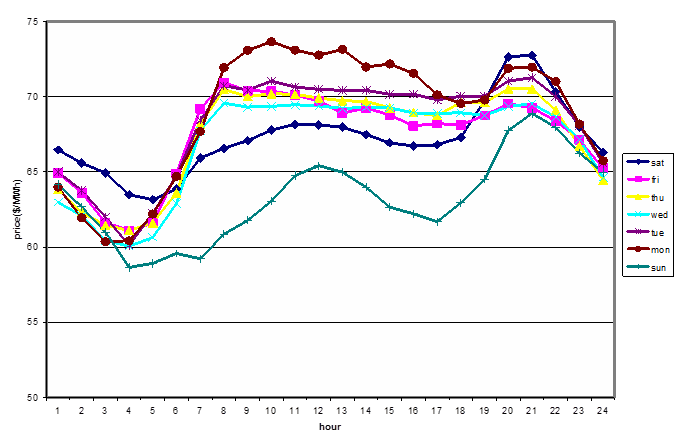Figure 1. Relationship of price and the days of the week

#### 4.3. Sensitivity Analysis of the Weather Condition

To predict the loads, combination of weather factors like temperature, wind speed and cloudiness, and previous day’s prices have been used. As the result shown, the correlation between price and wind speed, and correlation between price and cloudiness is negligible, and can be ignored. However, temperature has a great effect on short-term prices prediction; lack of accurate temperature is a big problem in prediction. Therefore, for sensitivity analysis, the daily temperature and the average price per day have been considered. Figure 2 shows the price sensitivity to temperature.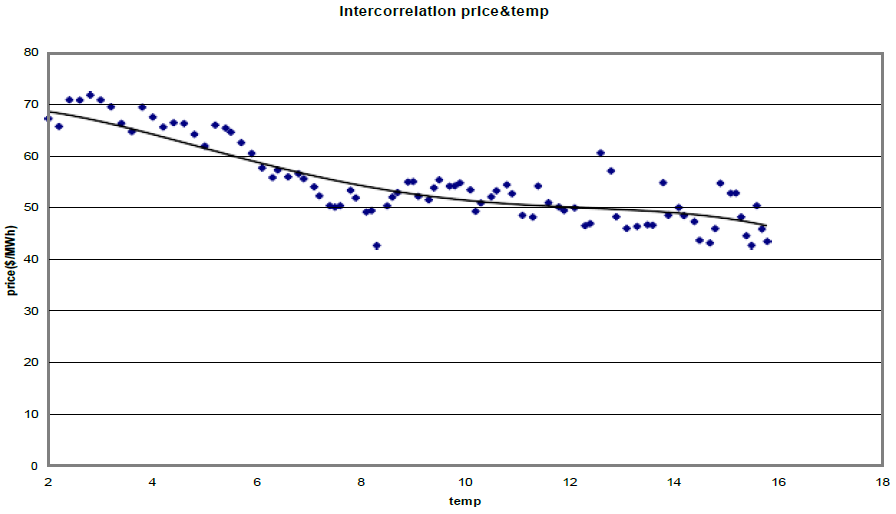Figure 2. Price sensitivity depend on Temperature

#### 4.4. Sensitivity Analysis of Hourly Price to the Price of Previous Hours

To find dependence of hourly price to previous price-hour, correlation factors between each hour and 1 to 168 previous hours (a week) are computed. Correlation diagram is shown in figure 3 and the correlation factor is 85%.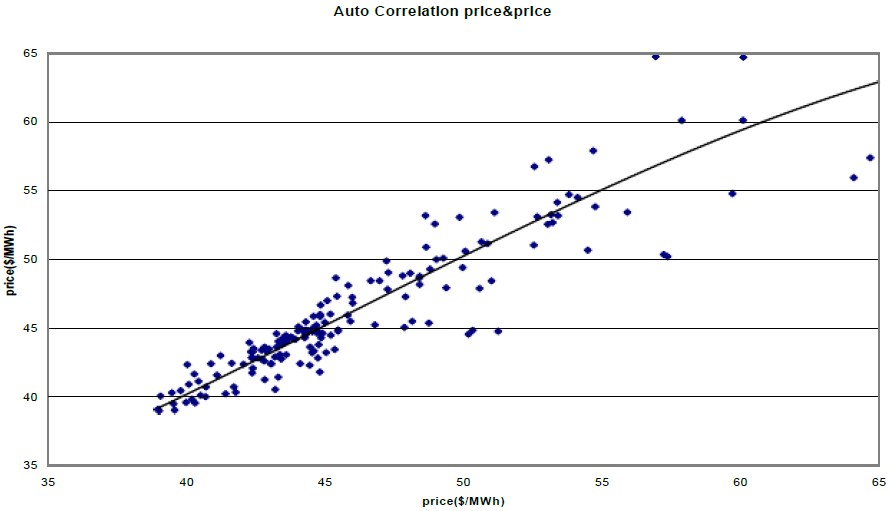Figure 3. Correlation between hourly prices with previous price of 1 to 168 hours

#### 4.5. Sensitivity Analysis of Electricity Price to the Load of Previous Hours

In this analysis, cross-correlation sensitivity analysis used for the hourly price differences and electricity load differences. This method causes the parameters to be uniformed. Figure 4 depicts sensitivity of electricity prices depend on previous amounts of system.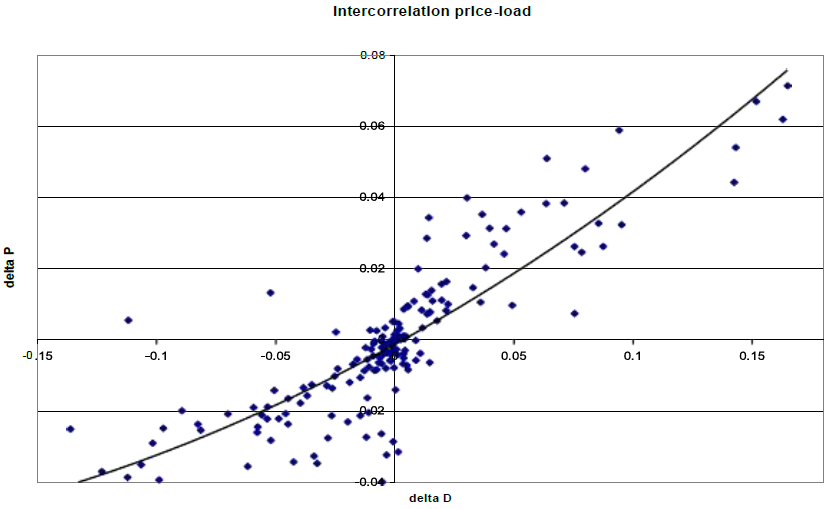Figure 4. Dependency of Prices sensitivity on previous amounts of load system

#### 5.1. Classification and Selection of Input Data

After performing sensitivity analysis on the input data, to find an appropriate model for classifying the neural networks input data, the consumption of weekdays and weekend has been considered. Table 1 shows the classification of weekdays and weekend based on the load pattern of figure 5.
Figure 5 shows the daily load pattern for one week in summer.
 Table 1. Classification of different days of a week based on the load pattern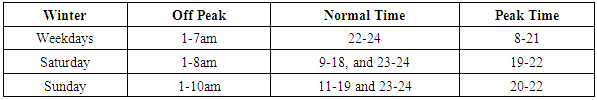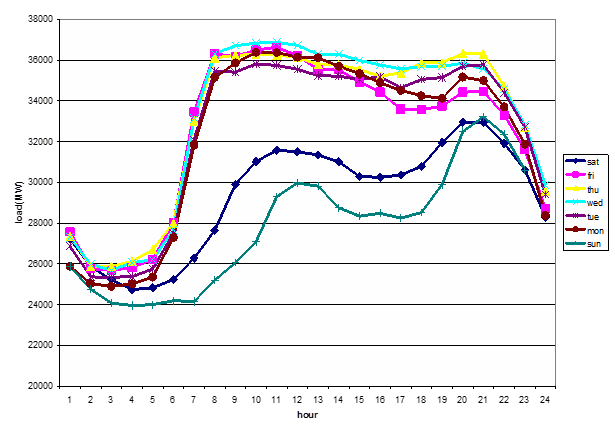Figure 5. Daily load consumption in summer

#### 5.2. Flow Chart of the Load Forecasting Process

The algorithm can be presented within the following processes:
1- Selecting data and preparing them
2- Sensitivity analyses of input data
3- Training process
4- Data scaling
5- Obtaining network’s parameters
6- Forecasting process
7- Simulation and extracting hourly forecast’s results
8- Calculating forecast’s errors
Figure 6 shows the algorithm of load forecasting process.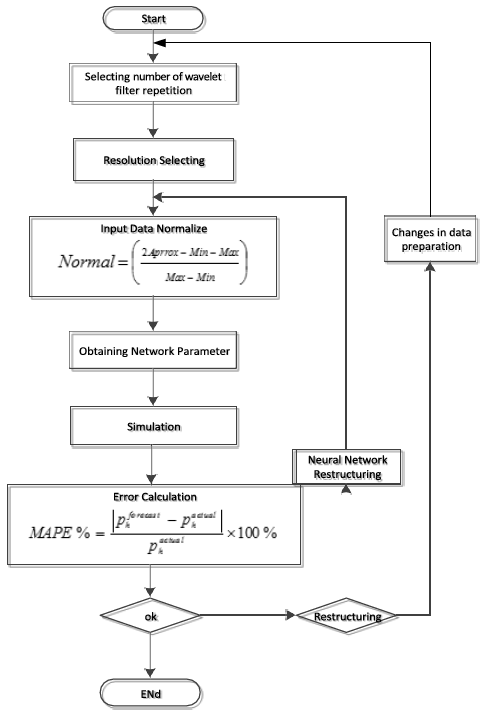Figure 6. Proposed load forecasting process algorithm

### 6. Price Forecasting

Forecasting can be done separately for each 1 to 168 hour. Initially the price of first hour is calculated, and then the price is used to predict the next hours. Also, since there is only one input vector for each hour, for scaling the inputs and converting the forecast results from scaled mode to actual mode, the maximum and minimum amount of input and output are needed. So, based on previous price, the maximum and minimum vectors of the input and output will be obtained. Then, the Mean Absolute Percent Error is calculated.

The proposed model is applied to Nord Pool market for testing the accuracy of forecast. Using wavelet functions and a filter can increase the accuracy of prediction and reduce the price forecasting disorders. Figure 7 shows the load signal, approximation signal and details of wavelet transform in Nord Pool Market.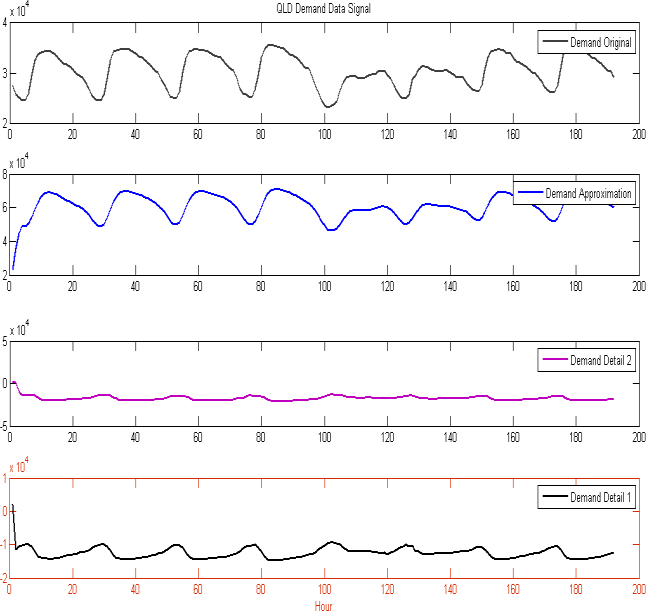Figure 7. Load signal, approximation signal and details of Wavelet transform

#### 6.2. Price Prediction Model

Overall structure of this method is not limited to one neural network; the number of neural networks is equal to: the number of resolutions +1. As it can be seen in figure 8, to increase accuracy and reduce the disorders, wavelet function with a filter has been used. Therefore, two training signals come from this filter; first with low changes and second with high changes. After training the neural networks, the results of each stage will be combined by a reverse- wavelet filter again, and the original output signal will be produced.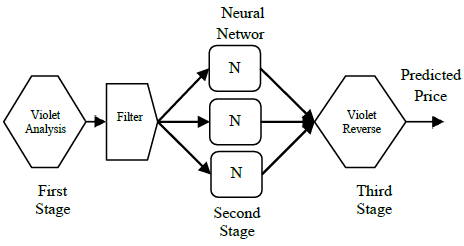Figure 8. Price Prediction Model

### 7. Results and Discussions

#### 7.1. Results in Summer

The proposed method was tested on Nord Pool electricity market and the results of each season obtained in following three stages:
1. Considering hour and day for each data and its electricity charge
2. Considering hour and day for each data, price, electricity charge, and temperature
3. Considering hour and day for each data, price, predicted electricity charge, and temperature
These data were selected from August to September, and analyzed in three stages. According to consumption patterns derived from the data in figure 9, table 2 will be obtained for consumption pattern.
Table 2 shows the consumption pattern in summer according to day and hour of September 2016.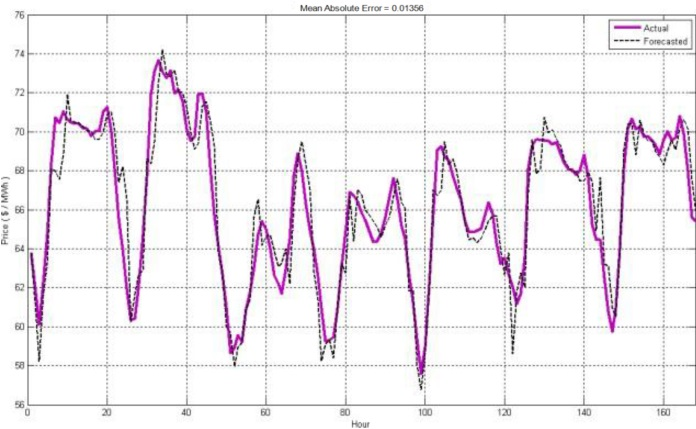Figure 9. Daily consumption in Nord pool market at September 2016
 Table 2. Consumption pattern according to day and hour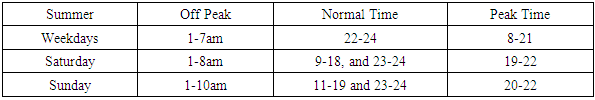##### 7.1.1. First Stage
The network structure consists of three inputs including day and hour, hourly price, and electric load corresponding to each hour of price, and two resolutions and four days data selected for data categorizing. 300 time iteration are considered for training and three hidden layers 19, 19, and 19 used for each applied neural network in training and forecasting process. Figure 10 depicts the price forecasting for a week in Nord Pool market (September 2016). As the results show the mean absolute percentage error in this stage is 1.76%.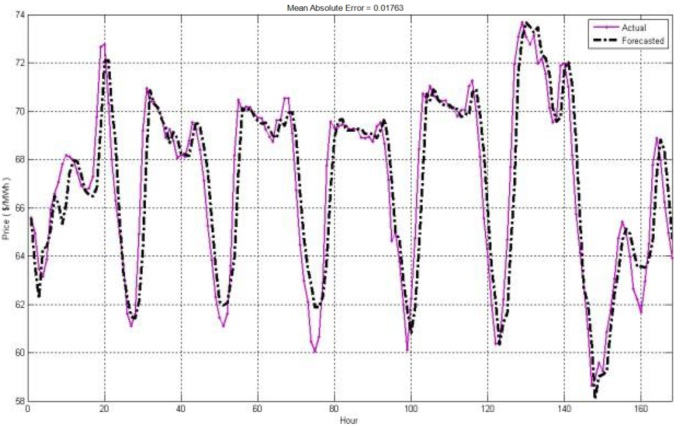Figure 10. Price forecasting for one week at September 2016
##### 7.1.2. Second Stage
The network structure consists of four inputs including day and hour, hourly price, electricity load and the temperature corresponding to each hour of price. The data categorizing of neural network input consists three resolutions and data of four days. 200 times iteration is considered for training and three hidden layers 31, 35, and 37 used for each applied neural network in training and forecasting process. Figure 11 shows the price forecasting in comparison by actual price for one week (September 2016) in Nord Pool market. As the results shows the mean absolute percentage error in this stage is 1.35%.
Table 3 compares the mean absolute percentage error with and without considering temperature in summer in Nord Pool market and comparison with previous stage.Figure 11. Price forecasting with considering temperature at September 2016
 Table 3. Mean absolute percentage error in Nord Pool market for one week at September 2016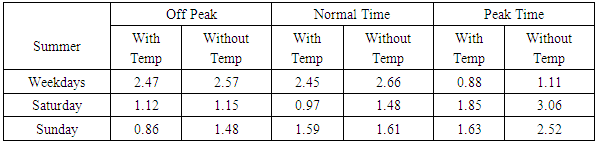As can be seen from table 3 and figure 11, the mean absolute percentage error by considering the temperature is lower than without considering temperature. Indeed, the second stage realizes a reduction of price prediction error due to considering temperature.
##### 7.1.3. Third Stage
In this stage, the inputs of network structure consist of hourly price, predicted electric load and temperature corresponding to each hour of price. The data categorizing of neural network input consists two resolution and data of one day. 300 times iteration is considered for training and three hidden layers 19, 17, and 20 used for each applied neural network in training and forecasting process. Table 4 compares the mean absolute percentage error at three stages separately in Nord Pool market (September 2016).
 Table 4. Mean absolute percentage error in Nord Pool market for one week at September 2016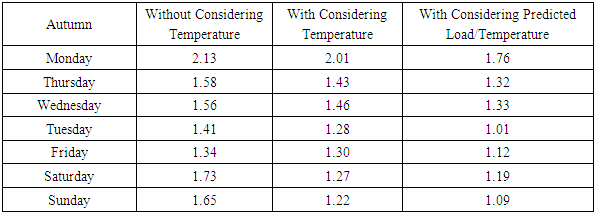#### 7.2. Result in Winter

In this season, the data were selected from February to march and have been investigated in three mentioned stages. According to the consumption pattern in figure 12, table 5 will be obtained for consumption pattern.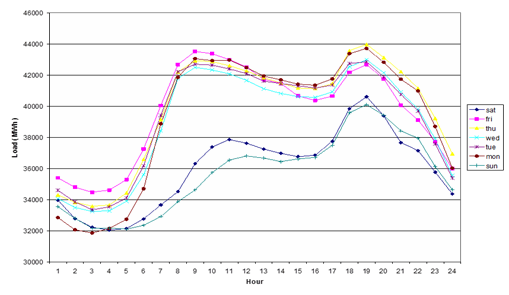Figure 12. Daily consumption in Nord pool market at February 2016
 Table 5. Consumption pattern according to day and hour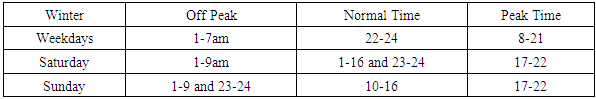Table 5 shows the consumption pattern in winter according to day and hour.
##### 7.2.1. First Stage
Three inputs consist of day and hour, hourly price, and electric load corresponding to the data. The two resolutions and seven days data selected for data categorizing. 200 times iteration is considered for training and three hidden layers 17, 17, and 19 used for each applied neural network in training and forecasting process. Figure 13 shows the results of price forecasting in comparison by actual price in Nord Pool market for one week at March 2016. As the result shows the mean absolute percentage error in this stage is 2.66%.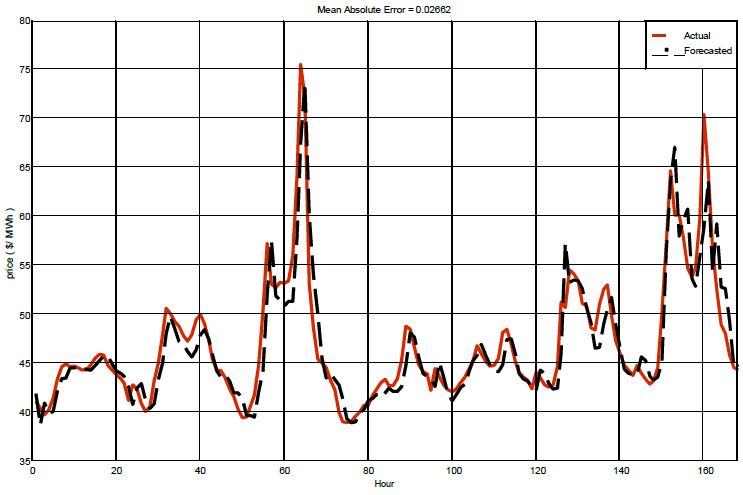Figure 13. Price forecasting without considering temperature at March 2016
##### 7.2.2. Second Stage
In this stage the four inputs consist of day and hour, hourly price, electric load and temperature corresponding to each hour of price, and the three resolutions and four days data for data categorizing. 200 times iteration is considered for training and four hidden layers, 19, 17 and 19 used for each applied neural network in training and forecasting process. Table 6 shows mean absolute percentage error of electricity price forecasting for each week (December 2016) in Nord Pool market, also results compared with first stage.
 Table 6. Mean absolute percentage error for a week at March 2016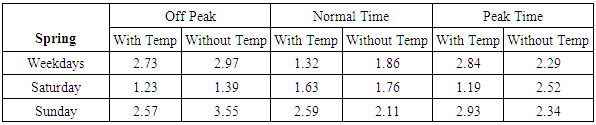Figure 14 shows price forecasting in comparison by actual price with considering temperature in Nord Pool market for one week at March 2016. As the results reveal the mean absolute percentage error in this stage is 2.14%.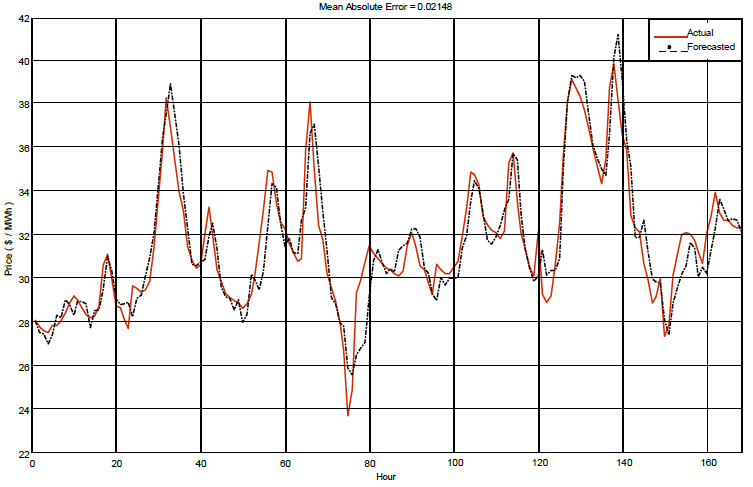Figure 14. Price forecasting with considering temperature at March 2016
##### 7.2.3. Third Stage
In this stage, four inputs consist of day and hour, hourly price, electric load and temperature corresponding to these data, and three resolution and four days selective data for data categorizing. 200 times iteration is considered for training and two hidden layers 15, 19 used for each applied neural network in training and forecasting process. Table 7 compares the mean absolute percentage error at three stages separately in Nord Pool market for each week (December 2016).
 Table 7. Mean absolute percentage error in Nord Pool market for one week at December 2016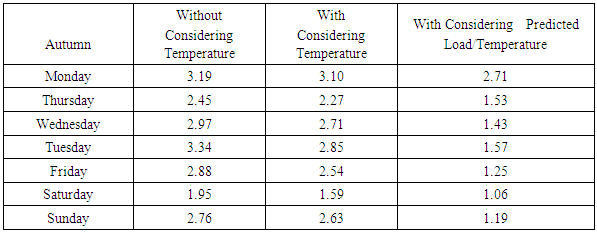#### 7.3. Result in Spring

This season data have been selected from June to May and have been investigated in three mentioned stages. According to consumption pattern of this data, table 8 will be obtained of consumption pattern.
 Table 8. Consumption pattern according to day and hour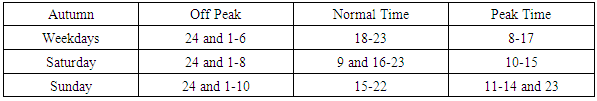##### 7.3.1. First Stage
In the first stage the three inputs consist of day and hour, price of each hour and electric charge corresponding to hour; also, two resolutions and four days selected for data categorizing. 200 times iteration is considered for training and three hidden layers 19, 19, 19 used for each applied neural network in training and forecasting process. The figure 15 shows the price forecasting in comparison by actual price in Nord Pool market for one week at June 2016. As the result shows the mean absolute percentage error in this stage is 4.73%.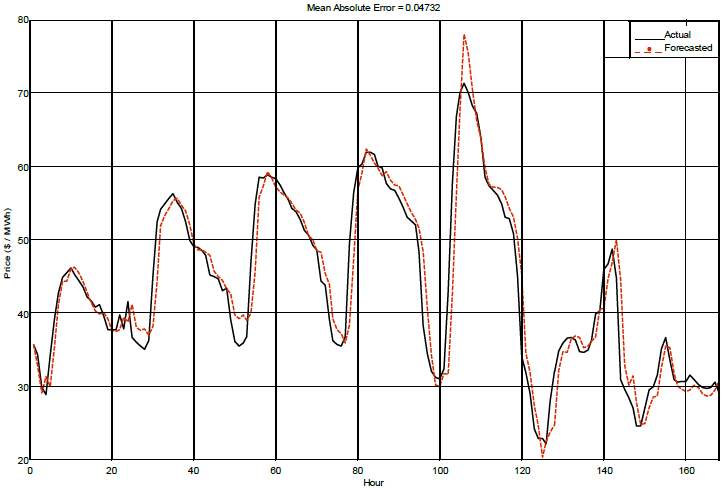Figure 15. Price forecasting at June 2016
##### 7.3.2. Second Stage
The four inputs in this stage consist of day and hour, hourly price, and electric load corresponding to each hour; also, the two resolutions and one day data selected for categorizing. 500 times iteration is considered for training and three hidden layers 19, 17, 20 used for each applied neural network in training and forecasting process. Table 9 presents the mean absolute percentage error of electricity price forecasting for each week (June 2016) in Nord Pool market, also results compared with first stage.
 Table 9. Mean absolute percentage error for one week at June 2016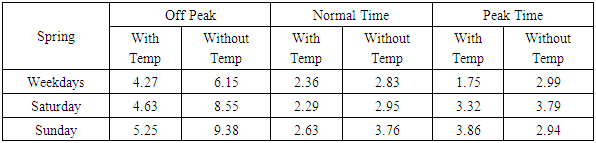##### 7.3.3. Third Stage
In this stage four inputs consist of day and hour, hourly price, and electric load corresponding to each hour of price, and two resolutions and one day data selected for data categorizing. 200 times iteration is considered for training and two hidden layers 19, 17 used for each applied neural network in training and forecasting process. Table 10 compares the mean absolute percentage error at three stages separately in Nord Pool market for each week (June 2016).
 Table 10. Mean absolute percentage error for one week at June 2016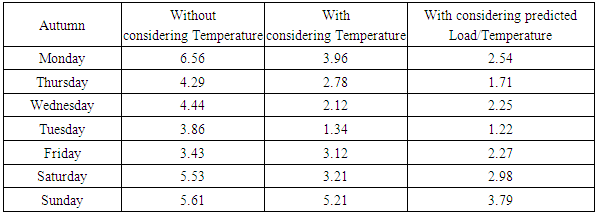#### 7.4. Result in Autumn

Data in this season have been selected from December and November and investigated in three stages. According to consumption pattern from this data in figure 16, table 11 will be obtained for consumption pattern.
Table 11 shows the consumption pattern in autumn according to day and hour.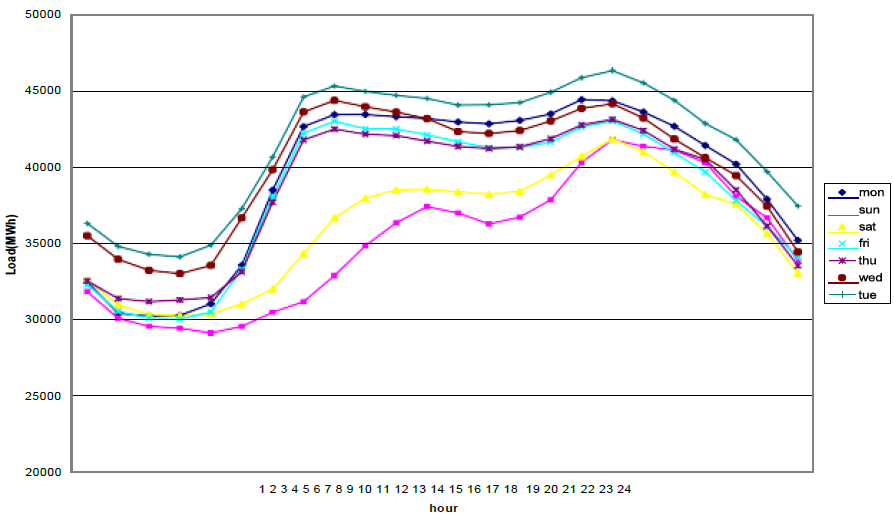Figure 16. Weekly consumption in Nord pool market at December 2016
 Table 11. Autumn consumption patterns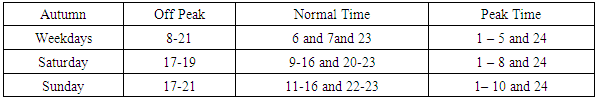##### 7.4.1. First Stage
At first stage the three inputs consist of day and hour, hourly price and electric charge corresponding to hour, and two resolutions and 4 days selection of data for data categorizing. 200 times iteration is considered for training and three hidden layers 19, 17 and 19 used for each applied neural network in training and forecasting process. Figure 17 shows the results of price forecasting in comparison by actual price for one week in Nord Pool market. The mean absolute percentage error in this stage is 3. 78%.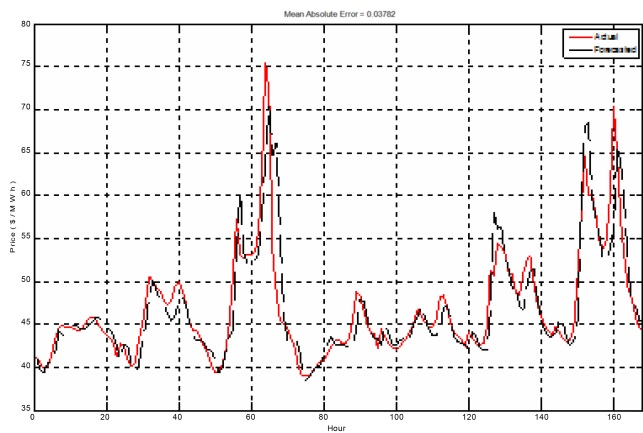Figure 17. Price forecasting for one week at December 2016
##### 7.4.2. Second Stage
In second stage, the four inputs consist of day and hour, hourly price and electric load corresponding to that hour, also, three resolutions and four days data selected for data categorizing. 300 times iteration is considered for training and three hidden layers 19, 17 and 19 used for each applied neural network in training and forecasting process. Table 12 compares the mean absolute percentage error for one week (December 2016) in Nord Pool market.
 Table 12. Mean absolute percentage error for one week at December 2016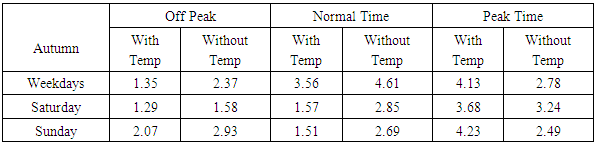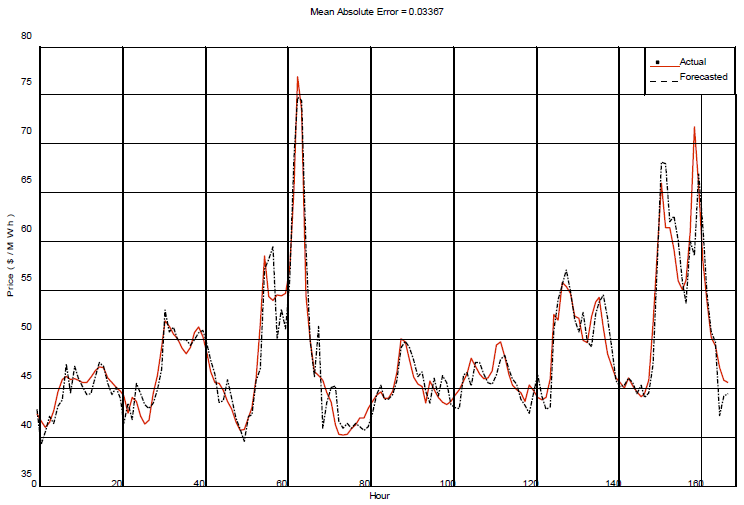Figure 18. Price forecasting for one week at December 2016
Figure 18 depicts results of price forecasting in comparison by actual price for next week (December 2016) in Nord Pool. As the result shows the mean absolute percentage error in this stage is 3.36%.
##### 7.4.3. Third Stage
In this stage the four inputs consist day and hour, hourly price, and electric load corresponding to each hour of price, and two resolutions and one day data selected for data categorizing. 300 times iteration is considered for training and two hidden layers 15, 17 used for each applied neural network in training and forecasting process.
 Table 13. Mean absolute percentage error for one week at December 2016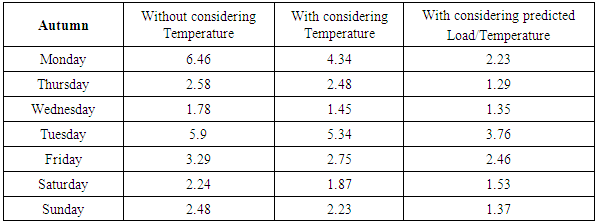The mean absolute percentage error of forecasting in Nord Pool market for one week at December 2016 is presented in table 13.

### 8. Conclusions

The proposed method has been tested on Nord Pool electricity market for each season separately, and extracted in following three stages.
1. Considering hour and day for each data and its electricity charge
2. Considering hour and day for each data, price, electricity charge, and temperature
3. Considering hour and day for each data, price, predicted electricity charge, and temperature
As the results show, forecasting results have a great influence on the energy market. Also consumers who have different production needs able to plan their production based on the results of price forecasting and escape from the very high prices of spot markets. In this paper, neural networks used for short time price forecasting because of its particular ability to discover non-linear relationship between the data. Also using consumption curves of each season, a separated model was obtained for Nord Pool market. The proposed method has been tested on Nord Pool market and the accuracy of results obtained using mean absolute percent error (% MAPE). To reduce the size of the input data to the neural network and to obtain better results, a filter used to separate parameters with similar frequency.
Furthermore, to achieve better results of input, in addition to the electrical loads, temperature has been used as a factor for forecasting. According to the result of various stages forecasting, by adding suitable input parameters the forecasting absolute percentage error would be reduced.

### References

  J. W. Taylor, L. M. de Menezes, and P. E. McSharry, "A comparison of univariate methods for forecasting electricity demand up to a day ahead," International Journal of Forecasting, vol. 22, pp. 1-16, 2006.  F. J. Nogales, J. Contreras, A. J. Conejo, and R. Espínola, "Forecasting next-day electricity prices by time series models," Power Systems, IEEE Transactions on, vol. 17, pp. 342-348, 2002.  J. Contreras, R. Espinola, F. J. Nogales, and A. J. Conejo, "ARIMA models to predict next-day electricity prices," Power Systems, IEEE Transactions on, vol. 18, pp. 1014-1020, 2003.  N. Kandil, R. Wamkeue, M. Saad, and S. Georges, "An efficient approach for short term load forecasting using artificial neural networks," International Journal of Electrical Power & Energy Systems, vol. 28, pp. 525-530, 2006.  V. Vahidinasab, S. Jadid, and A. Kazemi, "Day-ahead price forecasting in restructured power systems using artificial neural networks," Electric Power Systems Research, vol. 78, pp. 1332- 1342, 2008.  C. P. Rodriguez and G. J. Anders, "Energy price forecasting in the Ontario competitive power system market," Power Systems, IEEE Transactions on, vol. 19, pp. 366-374, 2004.  H. Y. Yamin, "Spinning reserve uncertainty in day-ahead competitive electricity markets for GENCOs," Power Systems, IEEE Transactions on, vol. 20, pp. 521-523, 2005.  Y. Y. Hong and C.-Y. Hsiao, "Locational marginal price forecasting in deregulated electric markets using a recurrent neural network," in Power Engineering Society Winter Meeting, 2001. IEEE, 2001, pp. 539-544.  D. W. Bunn, "Forecasting loads and prices in competitive power markets," Proceedings of the IEEE, vol. 88, pp. 163-169, 2000.  A. Khotanzad, E. Zhou, and H. Elragal, "A neuro-fuzzy approach to short-term load forecasting in a price-sensitive environment," Power Systems, IEEE Transactions on, vol. 17, pp. 1273-1282, 2002.  T. Peng, "Bidding strategy and empirical analysis of bidding in electrical power market," Washington State University, 2006.  F. Gao, X. Guan, X.-R. Cao, and A. Papalexopoulos, "Forecasting power market clearing price and quantity using a neural network method," in Power Engineering Society Summer Meeting, 2000. IEEE, 2000, pp. 2183-2188.